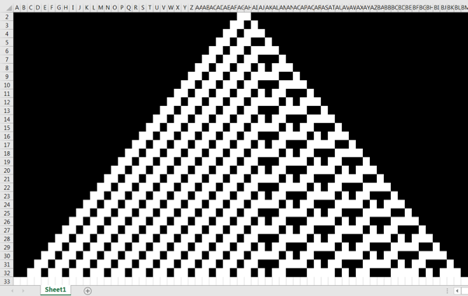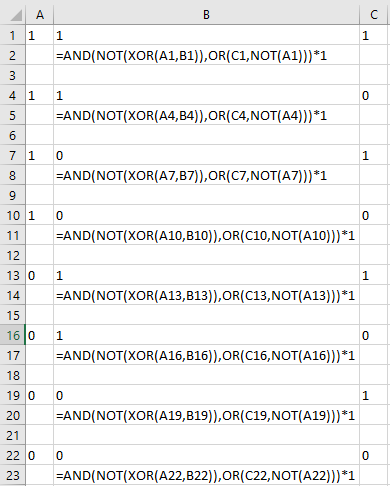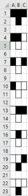## Cellular Automata in SQL Server

Feb 28

Written by: Charles Flock
2/28/2020 4:44 PMBased on Stephen Wolfram’s elementary cellular automata we demonstrate a very simple, straightforward approach to generating the cells for all 256 rules.

About 2 years ago I was watching a video of an MIT lecture on AGI (Artificial General Intelligence) given by Stephen Wolfram. He made several refences during the course of the lecture to his development of elementary cellular automata. During the course of the lecture, I thought it would be interesting to implement cellular automata in Excel without using VBA. Ultimately, that led to trying to do the same thing in SQL Server.

Here’s the output from Excel for rule 131 (there are 256 rules numbered from 0 to 255).According to Wolfram Alpha, Rule 131 can be expressed with the following operation:

(p, q, r) ↦ (NOT (p XOR q)) AND (r OR (NOT p))

and not knowing any better, I used this logic and some conditional formatting to come up with the Excel solution. As these are elementary cellular automata, the logic is actually pretty simple. Each row in the Excel worksheet is based on the previous row. The p, q and r values are the Left, Center and Right cells from the previous row. The only value is the cells are 0 or 1. We end up with something that looks like this:We can then apply some conditional formatting so that cells containing 1 are black and cells containing 0 are white. This results in the following representation of rule 131 in Excel.Here’s a way of implementing that logic in SQL Server:

SELECT
x
,~(p^q)&(r|~p) as val
FROM (
SELECT
CAST(x as CHAR(3)) as x
,CAST(SUBSTRING(x,1,1) as bit) as p
,CAST(SUBSTRING(x,2,1) as bit) as q
,CAST(SUBSTRING(x,3,1) as bit)as r
FROM (
SELECT
wct.DEC2BIN(SeriesValue,3) as x
FROM
wct.SeriesInt(7,0,-1,NULL,NULL)
)n
)p

This produces the following result for Rule 131.

All that remains is to convert the bit values into either dark spaces or white spaces. In the following SQL I am using the Unicode Full Block character and space to do that.

SELECT
x
,~(p^q)&(r|~p) as val
,CAST(REPLACE(REPLACE(x,'1',NCHAR(9608)),'0',SPACE(1)) as NCHAR(3)) as x
,CAST(REPLACE(REPLACE(~(p^q)&(r|~p),'1',NCHAR(9608)),'0',SPACE(1)) as NCHAR(1)) as val
FROM (
SELECT
CAST(x as CHAR(3)) as x
,CAST(SUBSTRING(x,1,1) as bit) as p
,CAST(SUBSTRING(x,2,1) as bit) as q
,CAST(SUBSTRING(x,3,1) as bit)as r
FROM (
SELECT
wct.DEC2BIN(SeriesValue,3) as x
FROM
wct.SeriesInt(7,0,-1,NULL,NULL)
)n
)p

This produces the following result for Rule 131.

At this point, I paused in my thinking. Wolfram calls these ‘elementary cellular automata’. This seemed a little harder than elementary. It seemed like I would have to code a different combination of bit-wise operation for each rule; 256 rules. That seemed like a lot of work.

I tried coming at the problem from a different angle. I wondered why it was called Rule 131. The following SQL reveals the answer to that question.

SELECT
CAST(wct.BIN2DEC(STRING_AGG(val,
'') WITHIN GROUP (
ORDER BY x DESC)) as tinyint) as [Rule]
FROM
(
SELECT
x ,
~(p^q)&(r |~ p) as val
FROM
(
SELECT
CAST(x as CHAR(3)) as x ,
CAST(SUBSTRING(x, 1, 1) as bit) as p ,
CAST(SUBSTRING(x, 2, 1) as bit) as q ,
CAST(SUBSTRING(x, 3, 1) as bit)as r
FROM
(
SELECT
wct.DEC2BIN(SeriesValue,
3) as x
FROM
wct.SeriesInt(7,
0,
-1,
NULL,
NULL) )n )p )q

This produces the following result.

In binary, the value of 131 is expressed as 10000011, the exact pattern we see returned from our first SQL statement. All the rules work this way. And, if we treat the binary representation of the rule as a string, we can simply search the string to get the correct value. However, since 111 (decimal value 7) returns the left-most value and 000 (decimal value 0) returns the right-most value, the simplest thing to do is reverse the string. I used the following SQL to generate the return values for Rule 131.

SELECT
CAST(wct.DEC2BIN(k.seriesValue,
3) as char(3)) as binval ,
SUBSTRING(REVERSE(wct.DEC2BIN(131, 8)), k.SeriesValue + 1, 1) as binrule
FROM
wct.SeriesInt(7,
0,
-1,
NULL,
NULL)k

This returns the following result:

This approach works for every Rule. In the following SQL we generate all the possible outcomes for Rules 0 to 255 and pivot the results to make it easier to read.

DECLARE @ColumnName as nvarchar(max);
DECLARE @DynamicPivot as nvarchar(max);

CREATE TABLE #CA ( binval char(3) ,
numrule int ,
binrule char(1) ,
PRIMARY KEY (binval,
numrule) );

INSERT
INTO
#CA
SELECT
wct.DEC2BIN(k.seriesValue,
3) as binval ,
l.seriesvalue as numrule ,
SUBSTRING(REVERSE(wct.DEC2BIN(l.seriesvalue, 8)), k.SeriesValue + 1, 1) as binrule
FROM
wct.SeriesInt(0,
7,
NULL,
NULL,
NULL)k CROSS APPLY wct.SeriesInt(0,
255,
NULL,
NULL,
NULL)l;

--Create Dynamic PIVOT
SELECT
@ColumnName = ISNULL(@ColumnName + ',',
'') + QUOTENAME(SeriesValue)
FROM
wct.SeriesInt(0,
255,
NULL,
NULL,
NULL);

--Prepare the PIVOT query
SET
@DynamicPivot = N'SELECT binval, ' + @ColumnName + '
FROM
#CA
PIVOT(MAX(binrule) For numrule IN (' + @ColumnName + ')) as pvt
ORDER BY binval DESC';

--Execute the dynamic pivot

EXEC sp_executesql @DynamicPivot;

This produces the following result.

Now, we are ready to write some SQL. We will use a simple initial condition: all zeroes except for the center value which is set to 1. We can think of the length of that string as the size, and we can think of the number of evolutions as the steps. In this particular approach, each row will be a step which consists of 2*@steps columns. And, each row is generated based on the previous row.

We will create a temporary table (#ca) to store the left (L), center (C) and Right (R) values from the previous row as well as the value to be generated for the Rule. All values are converted to binary and stored in the table. We create a table (#grid) to store the ‘evolution’.

The #grid table is in 3rd-normal form and is keyed by row (r) and column (c). We use the LAG function to get the left value and the LEAD function to get the right value. There are some decisions to made about calculations at the boundaries (when column = 1 or column = s * @steps + 1); you can see this in the default values for the LEAD and LAG functions. Additionally, I have arbitrarily set column 1 to 1 in all cases, simply to maintain consistency with the Wolfram Alpha example and the Excel implementation.

I use the Results to Text option in SSMS and then shrink the size of the output.

DECLARE @rule as int = 131;

SET
NOCOUNT ON;

--Table to store the (L)eft, (C)enter, (R)ight and Rule values
CREATE TABLE #ca ( L int ,
C int ,
R int ,
binrule CHAR(1) ,
Primary Key(L,
C,
R) );

--Generate the values for this rule
INSERT
INTO
#ca
SELECT
SUBSTRING(wct.DEC2BIN(k.seriesValue, 3), 1, 1) as L ,
SUBSTRING(wct.DEC2BIN(k.seriesValue, 3), 2, 1) as C ,
SUBSTRING(wct.DEC2BIN(k.seriesValue, 3), 3, 1) as R ,
SUBSTRING(REVERSE(wct.DEC2BIN(@rule, 8)), k.SeriesValue + 1, 1) as